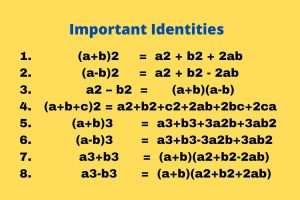Join Our WhatsApp Group!

# Polynomial for class 10 – Amazing Concepts & Notes PDF

Let’s start with the explanation of polynomial for class 10 Concepts. Read all the below concepts and download the pdf of polynomial notes and solve all NCERT questions solution.

Now discuss the concepts of the polynomial for class 10.

## Polynomials

An expression of the form p(x) = a0+a1x+a2x2+……+anxn, where an is not equal to zero, is called a polynomial in x of degree n.

Some important identity or polynomial for class 10 formulas used in polynomial:### Degree of polynomials

If P(x) is a polynomial in x, the highest power of x in P(x) is called the degree of the polynomial P(x).

Ex: The degree of polynomial P(x) = 2x3 + 5x2 -7 is 3 because the degree of the polynomial is the highest power of polynomial.

### Types of Polynomials

1. Linear polynomial: Polynomial having degree 1 is called a linear polynomial. For example, (4x+7), (3x-4), etc.

1. Quadratic polynomial: Polynomial having degree 2 is called a quadratic polynomial. For example, 2x2+5x+3, 4x2-3x+4 etc.

1. Cubic polynomial: Polynomial having degree 3 is called a cubic polynomial. For example, 3x3+4x2+5x+2, 9x3+5x2+3x+5 etc.

1. Biquadratic polynomial: Polynomial having degree 4 is called a biquadratic polynomial. For example, 5x4+3x3+8x2+x+2 etc.

Zero of polynomial

If the value of P(x) at x = K is zero then K is called a zero of the polynomial P(x).

## Relationship between zeros and coefficients of a polynomial

1. For Quadratic equation: If α and β are zeros of the quadratic equation ax2+bx+c, a is not equal to zero, then
• α+ β = -b/a
• αx β = c/a

P(x) = αx2 – (α +β)x + xβ

1. For Cubic equation: If α, β and γ are zeros of cubic polynomial ax3+bx2+cx+d, a is not equal to zero, then
• α+ β + γ            = -b/a
• αβ + βγ + γα    = c/a
• αβγ                    = -d/a

Equation of cubic polynomial,

P(x) = α x3 – (α +β+γ)x2 + (α β+βγ+γα )x – αβγ

## Division Algorithm for polynomials

If P(x) and g(x) are any two polynomials with g(x) is not equal to zero, then we can find polynomials q(x) and r(x) such that

P(x) = g(x) X q(x) + r(x)

When r(x) = 0 or degree of r(x) < degree of g(x). This result is called the division algorithm for polynomials.

## Some important identities used in Trigonometry and polynomials chapter

There are 8 important identities which is used in Trigonometry and Polynomial chapter:

1. (a+b)2 = a2 + b2 + 2ab
2. (a-b)2 = a2 + b2 – 2ab
3. a2 – b2 = (a+b)(a-b)
4. (a+b+c)2 = a2+b2+c2+2ab+2bc+2ca
5. (a+b)3 = a3+b3+3a2b+3ab2
6. (a-b)3 = a3+b3-3a2b+3ab2
7. a3+b3 = (a+b)(a2+b2-2ab)
8. a3-b3 = (a+b)(a2+b2+2ab)

## Graphical representation of zero of a polynomial

1. The zeroes of polynomial P(x) are precisely the x-coordinates of the points, where the graph of y=P(x) intersects the x-axis.
2. A quadratic polynomial can have at most 2 zeros and a cubic polynomial can have at most 3 zeros.
3. For any quadratic polynomial ax2+bx+c, a is not equal to zero, its graph one of the two shapes either open upwards or open downwards on whether a>0 or a<0.

## Factorization is the important concept used in Polynomial

Factorization: The process of finding two or more factors of a given expression is called factorization.

EX: Find the factor of x2-4.

Solution: We know that a2-b2= (a+b)(a-b)

X2-4 = x2-22 = (x+2)(x-2)

Hence, (x+2) and (x-2) is the factor of x2-4.

Factorize x2+5x+6.

Solution: By using middle splitting term method,

= x2+5x+6

= x2+2x+3x+6

= x(x+2) +3(x+2)

= (x+2)(x+3)

Hence, (x+2) and (x+3) is the factor of x2+5x+6.

I hope you enjoy this post polynomial for class 10 all concept notes.

View more…